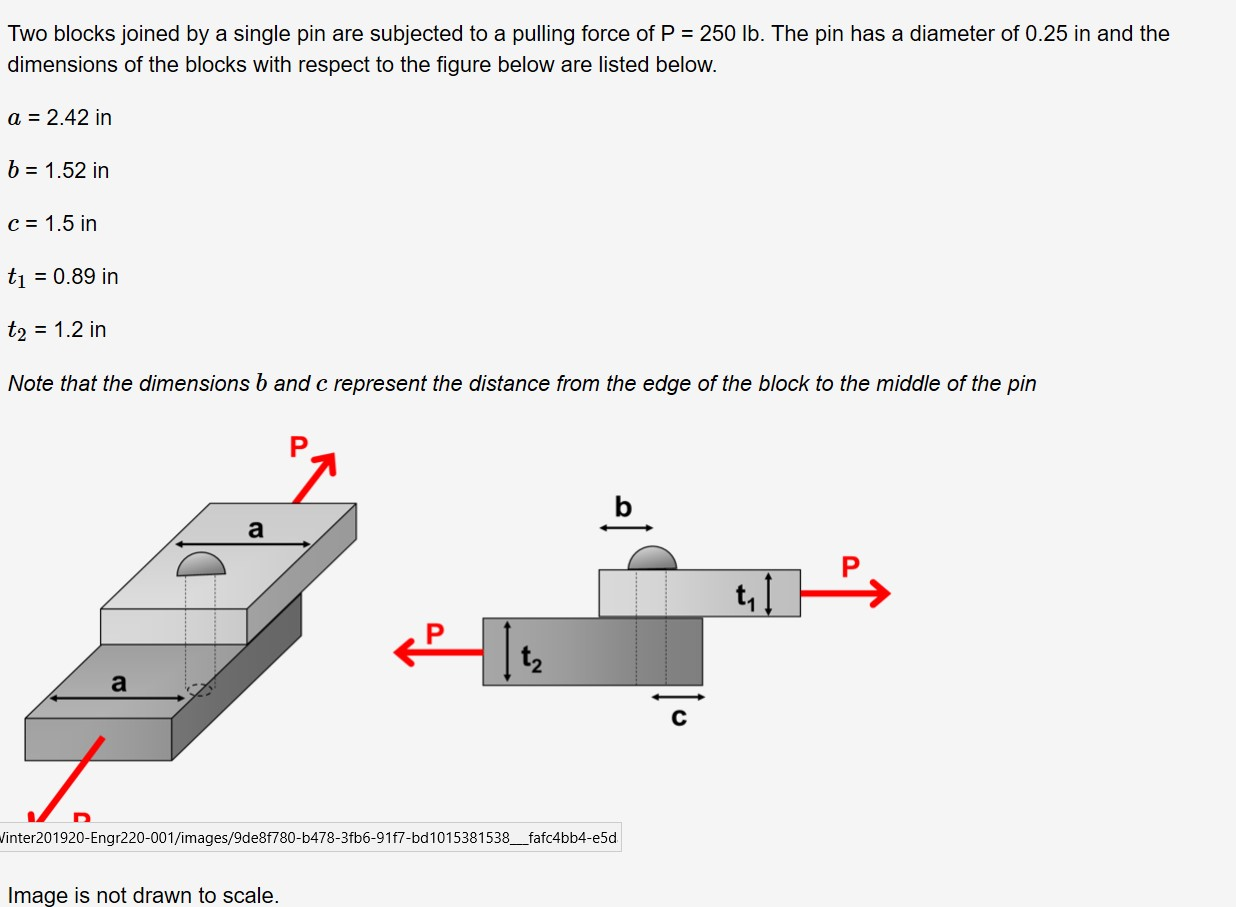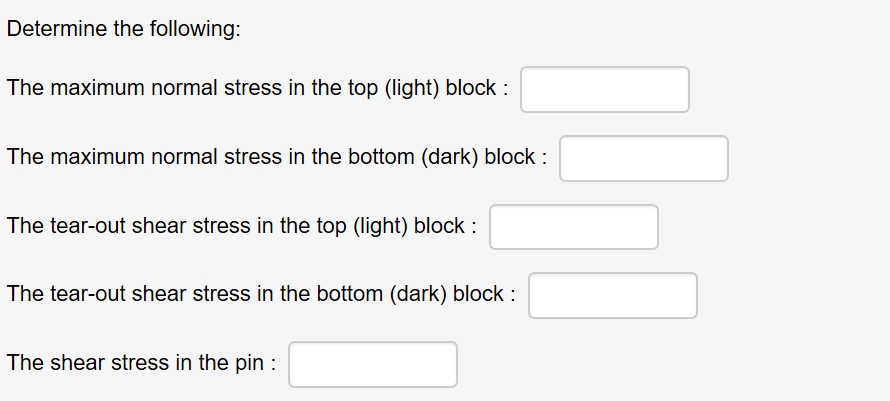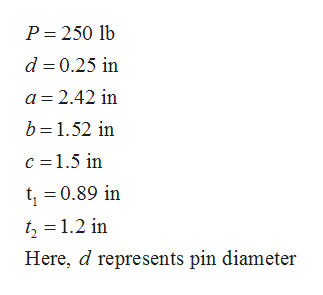# Two blocks joined by a single pin are subjected to a pulling force of P = 250 Ib. The pin has a diameter of 0.25 in and thedimensions of the blocks with respect to the figure below are listed below.a = 2.42 inb = 1.52 inC = 1.5 inti = 0.89 int2 = 1.2 inNote that the dimensions b and c represent the distance from the edge of the block to the middle of the pint, 1aVinter201920-Engr220-001/images/9de8f780-b478-3fb6-91f7-bd1015381538_fafc4bb4-e5dImage is not drawn to scale. Determine the following:The maximum normal stress in the top (light) block :The maximum normal stress in the bottom (dark) block :The tear-out shear stress in the top (light) block :The tear-out shear stress in the bottom (dark) block :The shear stress in the pin :

Question
6 viewshelp_outlineImage TranscriptioncloseTwo blocks joined by a single pin are subjected to a pulling force of P = 250 Ib. The pin has a diameter of 0.25 in and the dimensions of the blocks with respect to the figure below are listed below. a = 2.42 in b = 1.52 in C = 1.5 in ti = 0.89 in t2 = 1.2 in Note that the dimensions b and c represent the distance from the edge of the block to the middle of the pin t, 1 a Vinter201920-Engr220-001/images/9de8f780-b478-3fb6-91f7-bd1015381538_fafc4bb4-e5d Image is not drawn to scale. fullscreenhelp_outlineImage TranscriptioncloseDetermine the following: The maximum normal stress in the top (light) block : The maximum normal stress in the bottom (dark) block : The tear-out shear stress in the top (light) block : The tear-out shear stress in the bottom (dark) block : The shear stress in the pin : fullscreen
check_circle

Step 1

Since you have posted a question with multiple sub-parts, we will solve the first three subparts i.e. 1st, 2nd, and 3rd for you out of the 5 asked. To get the remaining sub-part (4th and 5th) solved, please re-post the question with the remaining sub-parts.

The given data are:help_outlineImage TranscriptioncloseP= 250 lb d = 0.25 in a = 2.42 in b = 1.52 in c =1.5 in t, = 0.89 in tz = 1.2 in Here, d represents pin diameter fullscreen
Step 2

To calculate the maximum normal stress in the top block, first calculate the minimum tensile area resisting the force P.

Step 3

Calculate the maximum normal s...

### Want to see the full answer?

See Solution

#### Want to see this answer and more?

Solutions are written by subject experts who are available 24/7. Questions are typically answered within 1 hour.*

See Solution
*Response times may vary by subject and question.
Tagged in
EngineeringMechanical Engineering

### Solid Mechanics